## Friday, 8 August 2014

### CHAPTER 16-Dot Product

We have already seen the addition and subtraction of vectors. In this section, we’ll understand how we can define the product of two vectors.
Before formally defining the dot product, let us try to understand why (such a) product is required at all.
Consider a force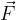$\vec F$ acting on a block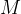$M$ at an angle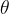$\theta$ to the horizontal.This block, as an effect of the force, is displaced through a horizontal distance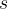$s$. We can denote the displacement by the vector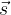$\vec s$, which has a horizontal direction and has a magnitude$s$.In physics, you must have studied the concept of the work done by a force. This work done is the maximum if the force and the displacement caused by it are in the same direction, and zero if the force and the displacement are perpendicular. This suggests that we must consider the component of$\vec F$ along$\vec s$ to evaluate its work done.
From the figure above, the component of$\vec F$ along the direction of$\vec s$ is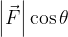$\left| {\vec F} \right|\cos \theta$. The work done will then be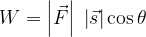$W = \left| {\vec F} \right|\;\left| {\vec s} \right|\cos \theta$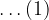$\ldots(1)$
We denote the right hand side of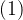$(1)$ by the dot product notation.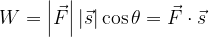${W = \left| {\vec F} \right|\left| {\vec s} \right|\cos \theta = \vec F \cdot \vec s}$
In general, for two vectors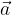$\vec a$ and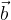$\vec b$ inclined at an angle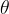${\rm{\theta }}$ to each other, their dot product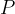$P$ is defined as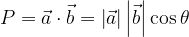$P = \vec a \cdot \vec b = \left| {\vec a} \right|\left| {\vec b} \right|\cos {\rm{\theta }}$
It is very important to understand that$P$ is a scalar quantity
You can think of$P$ in this way: it is a measure of the “effect” of one vector along the other. For two vectors of fixed magnitude , their dot product will decrease in magnitude as${\rm{\theta }}$ increases from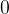$0$ to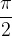$\dfrac{{\rm{\pi }}}{2}$ (or decreases from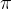$\pi$ to$\dfrac{\pi }{2}$ ).
We can write$P$ as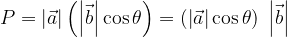$P = \left| {\vec a} \right|\left( {\left| {\vec b} \right|\cos \theta } \right) = \left( {\left| {\vec a} \right|\cos \theta } \right)\;\left| {\vec b} \right|$
Thus,$P$ is the product of the modulus of either vector and the projection of the other in its direction.